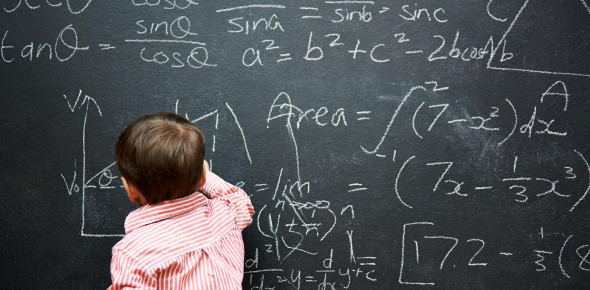# Dividing Decimals Practice Quiz

Approved & Edited by ProProfs Editorial Team
At ProProfs Quizzes, our dedicated in-house team of experts takes pride in their work. With a sharp eye for detail, they meticulously review each quiz. This ensures that every quiz, taken by over 100 million users, meets our standards of accuracy, clarity, and engagement.
| Written by De_fenestration
D
De_fenestration
Community Contributor
Quizzes Created: 5 | Total Attempts: 2,043
Questions: 10 | Attempts: 333SettingsHow strong are your division skills in math? Would you be able to pass this dividing decimals practice quiz? Yes, you read that correctly. Here, you will not be diving into normal numbers but numbers that are in decimals. We will see if you are able to answer most of the questions correctly or not. You are expected to answer these questions independently without using the calculator's help. Give your best, and have fun!

• 1.

### 2.  12)0.12

Explanation
The answer .01, 0.01 is obtained by rearranging the given numbers in ascending order.

Rate this question:

• 2.

### 4.  0.22) 0.0132

Explanation
The given answer is 0.06 and .06. This suggests that the number 0.0132 is being rounded to two decimal places, resulting in two different representations. Both 0.06 and .06 are valid ways to express the rounded value of 0.0132.

Rate this question:

• 3.

### 6.  3.18) 0.636

Explanation
The given answer is 0.2 and .2. These are both equivalent decimal representations of the same number, which is 0.2.

Rate this question:

• 4.

### 1/0.1

Explanation
The given expression is a division of 1 by 0.1. When dividing a number by a decimal, we can convert the decimal into a whole number by moving the decimal point to the right. In this case, moving the decimal point one place to the right in 0.1 gives us 1. Dividing 1 by 1 equals 1, so the answer to the expression 1/0.1 is 10.

Rate this question:

• 5.

### 8.  82.3)  823

Explanation
The given sequence consists of numbers 8, 82.3, and 823. The pattern followed is that each number is obtained by multiplying the previous number by 10. Therefore, starting with 8, when we multiply it by 10, we get 80. Then, adding 2.3 to 80, we get 82.3. Finally, multiplying 82.3 by 10 gives us 823. Hence, the next numbers in the sequence would be 8230, 82300, and so on, all obtained by multiplying the previous number by 10.

Rate this question:

• 6.

### 10.  0.5) 85

Explanation
The correct answer is 170, 170., 170.0. The answer is 170 because it is the whole number value that is given in the options. The answer is also 170. and 170.0 because they are decimal representations of the whole number 170.

Rate this question:

• 7.

### 4/0.4

Explanation
The given expression is a division problem, where 4 is divided by 0.4. When dividing a number by a decimal, we can multiply both the numerator and denominator by 10 to eliminate the decimal. By doing so, we get 40 divided by 4, which equals 10. Therefore, the answer to the expression 4/0.4 is 10.

Rate this question:

• 8.

### 12.  19.2) 4.416

Explanation
The given answer 0.23 and .23 are the decimal representations of the same number. They are equivalent and represent the fraction 23/100.

Rate this question:

• 9.

• 10.

### 16.  812 / 0.4

Explanation
When dividing 812 by 0.4, the result is 2030. This is because dividing any number by a decimal is the same as multiplying it by the reciprocal of that decimal. In this case, the reciprocal of 0.4 is 2.5. So, 812 multiplied by 2.5 equals 2030. Therefore, the correct answers are 2030, 2030., and 2030.0.

Rate this question:

Related TopicsBack to top
×

Wait!
Here's an interesting quiz for you.